<body><script type="text/javascript"> function setAttributeOnload(object, attribute, val) { if(window.addEventListener) { window.addEventListener('load', function(){ object[attribute] = val; }, false); } else { window.attachEvent('onload', function(){ object[attribute] = val; }); } } </script> <div id="navbar-iframe-container"></div> <script type="text/javascript" src="https://apis.google.com/js/plusone.js"></script> <script type="text/javascript"> gapi.load("gapi.iframes:gapi.iframes.style.bubble", function() { if (gapi.iframes && gapi.iframes.getContext) { gapi.iframes.getContext().openChild({ url: 'https://www.blogger.com/navbar.g?targetBlogID\x3d15925399\x26blogName\x3dPre-Cal+40S+(Winter+\x2706)\x26publishMode\x3dPUBLISH_MODE_BLOGSPOT\x26navbarType\x3dBLUE\x26layoutType\x3dCLASSIC\x26searchRoot\x3dhttps://pc4sw06.blogspot.com/search\x26blogLocale\x3den_US\x26v\x3d2\x26homepageUrl\x3dhttp://pc4sw06.blogspot.com/\x26vt\x3d8306270521480406005', where: document.getElementById("navbar-iframe-container"), id: "navbar-iframe" }); } }); </script>

## Wednesday, March 01, 2006

### Scribe 15. WHY ME

HELLO
Hey guys. It's me, Regine. Thanks Anh. *wink wink* Man, and on a double period too. Oh well. You probably won't understand this, but at least I attempted it. Anyways, here I go.......going I am...Starting soon....hold that thought. I'm hungry.

In the second period, Mr. K started off with notes in second period so we had to write in our Math Dictionaries.

Even Functions:

Graphically: A function is "even" if its graph is symmetrical about the y-axis.

These are even functions: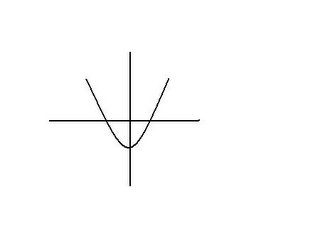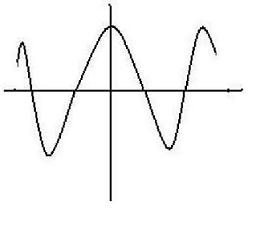These are not: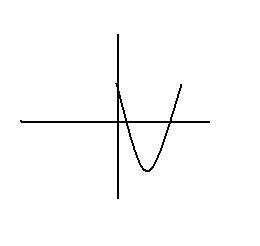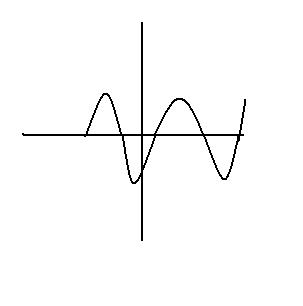Algebraically: A function is even iff f(-x)=f(x)
Examples: Are these functions even?

f
(x) = x^2 - 2x

f(-x) = (-x)^2 - 2(x)
= x^2 + 2x

This is not an even function
Therefore,
f(-x) =/= <----(cannot equal) f(x)

g
(x) = x^2 - x^4

g(-x) = (-x)^2 - (-x)^4

= x^2 - x^4

This is an even function
Therefore,
g(-x) = g(x)

ODD FUNCTIONS:

These functions are odd if the graph is symmetrical about the origin.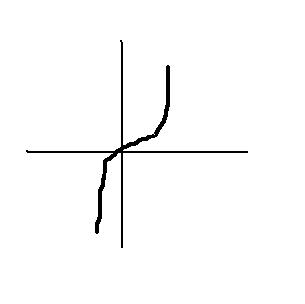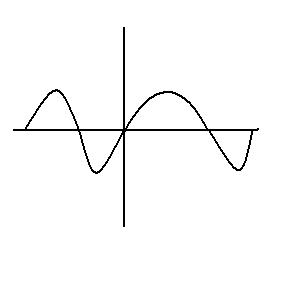These are not: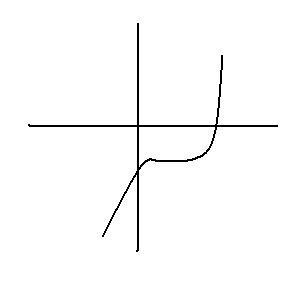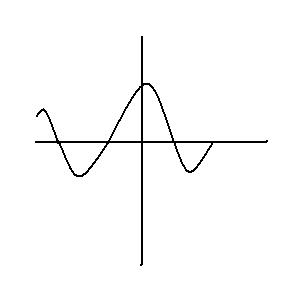Algebraically: A function is odd IFF f(x) = -f(x)
Examples: Are these functions odd?

f
(x) = x^3 - 4x

f(-x) = (-x)^3 - 4(-x)
= -x^3 + 4x

-f(x) = - (x^3 - 4x)
= -x^3 + 4x

f is odd
Therefore,
f(-x) = -f(x)

__________________

g(x) = x^2 - 3x

g(-x) = (-x)^2 - 3(-x)
= x^2 + 3x

-g(x) = - (x^2 - 3x)
= -x^2 + 3x

g is not odd
Therefore,
g(-x) =/= -g(x)

I like Lavender

Recap:

A function is even IFF its graph is symmetrical about the y-axis. If you reflect the graph onto itself, you should come up with an exact copy of its first half.

It is not a function if its reflection does not match its first half.

A function is odd if its graph is symmetrical about the origin. In other words, if you make a complete 180 degree turn, the graph should look like how it started. It's better to see a demonstration on paper. You know it is an odd function if you flip your paper around and it looks exactly the same.

In class, Mr. K had us write the reciprocals of these numbers

0 1 10 1000 1 000 000 1 000 000 000
Reciprocals Undefined 1 1/10 1/1000 1/1000 000 1/1 000 000 000

0 0.01 0.001 0.000 001 0.000 000 001
Reciprocals Undefined 10 1000 1 000 000 1 000 000 000

As whole numbers increase, their reciprocals decrease.
As decimal numbers decrease, their reciprocals increase.

Now, at this section. This is just Mr. K ranting about millions and trillions and british people and all that jazz. I looked mad through out this explaination.

How big is a billion?

British people call it a thousand millions. We call it a billion. Is it such a big difference?

If someone, let's say Manny were to be paid a dollar per second, how long would he have to sit in his desk until the amount reaches a billion?

Well if you take a billion and divide it by sixty, you'll get hours. If you take that answer and divide it by 24 hours, you'll end up with days. If you take that answer and divide it by 360, you'll end up with years.

Manny would have to sit in his desk for thirty two years. He can't do that because that's not humanly possible. He'll get multiple hemmerhoids from sitting for too long. That, students, is how big a billion is. It's a significant difference between the American and British billion.

Then. Mr. K mentioned the difference between a google and a googleplex. I'm getting tired so I"m not even going to mention this. And about the different types of infinities there are and there are infinite number of infinate infinity sets and I just started to get angry at the thought of this so I'm not going to bother to explain this either.

Next Section-4th Period

I'm going to try to explain this in one graph since I'm really tired and I need sleep..

So people, please bear with me and you probably stopped reading when you saw the word, "hello."

f(x) = x^2 - 4 Sketch:1/f(x)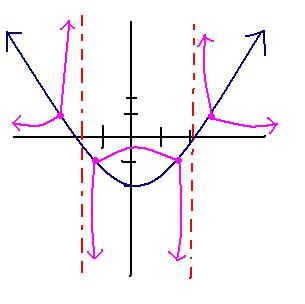The blue graph indicates f(x) = x^2 -4

To find the reciprocal out what the reciprocal of this graph looks like:

- You first draw your stated function (blue graph)

-Find the reciprocal of the function, y-intercept, and its roots. --> 1/(x+2)(x-2) roots:+/- 2
y intercept- (-1/4) <-- because the y intercept was -4. Take the reciprocal of that. - Use the roots to draw out where your asymptote(s)(am I spelling that right?) is/are. The red dashed line indicates where the asymptotes are.

- Draw out where your Invariants should be placed (which is 1 and -1). To do this, you look at the blue graph and place dots to where it meets 1 and -1. The purple dots on the graph above state where the invariant points are.

- Look at the right side of the blue graph. If the right side is biggering, then the one direction of your purple graph is smallering. Make sure the purple graph never touches the axes or the asymptotes. Check the left side of the blue graph, where the line is descending down. As that gets smaller, the other direction of the purple graph is. biggeringBOOM BAM. You do this for the rest of the graph. There you have it.
Hopefully, you understood some of that.

Maan. You won't get to see the other beautiful graphs I spent endless time on. Well. I'm proud of them so I'm posting them up. You basically follow what is stated above.

f(x) = x Sketch: 1/f(x)---------------------------------f(x) = x - 2 Sketch: 1/f(x)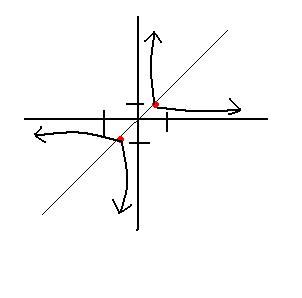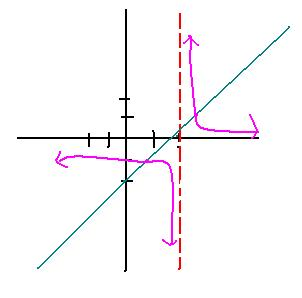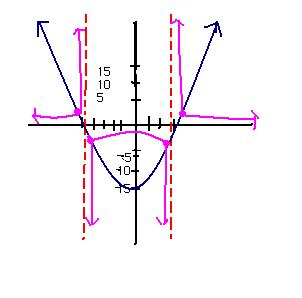F(x) = x^2 + 2x -15

Mr. K also a little about absolute values. I only remember the informal definition.

|5| |-5| <----Means how far the number within those enclosed lines are from 0. Absolute value of 5 is five units away from 0. Absolute number of -5 is five units away from zero. You can also express absolute values this way
|x| = x ; x>0
|x| = -x, ; x<0

f(x) = |x|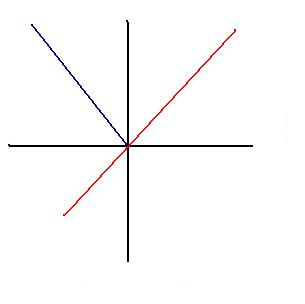The red line indicates the graph of x. But absolute values are all positive. therefore, you must flip the negative part of the graph into a positive sector. The blue line indicates where that positive sector is. The joined blue and red line on the positive sectors of this graph is the graph of absolute value of x.

I hope you understand this from just one graph. There two more, and they look awesome, so they'll just be posted up. It's the same explaination stated above. You just have to graph the stated function and make all its negative parts go through the positive sectors of the graph.

f(x) = x + 3 Sketch: |f(x)| ---------------------f(x) = x^2 - 4 Sketch: |f(x)|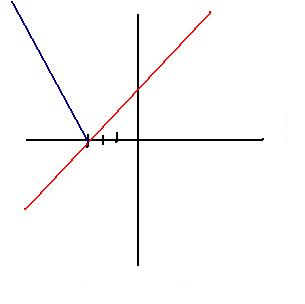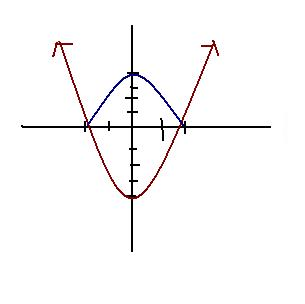AND I AM DONE. THIS TOOK TOO LONG AND I DID NOT ENJOY IT AT ALL. SO THE NEXT SCRIBE WILL BE JAN just because. He's smart. I like lavender

At 3/01/2006 3:04 PM,calvinw. said...

wow the scribes just keep growing and growing... ahhah its cool keep it growing, hopefully it doesnt take up the whole screen with the one post =( nice post

At 3/01/2006 5:50 PM,Jefferson said...

wow this is hardcore regine. good job. Mr K said your scribe was mind boggling and jaw dropping. He wasnt lying, you got more praise than me ;-) haha lawl, you covered everything

At 3/01/2006 6:16 PM,Anonymous said...

WOW!! that's loooong... wow.. wow... even after it got deleted.. wow... YAY

At 3/01/2006 9:09 PM,mark said...

lol it is a long scrie post and its ALOT! =D

At 3/02/2006 5:31 PM,Manny said...

i never got to use the computer till today because of time related issues... mr.k did say something about your post, and yeah, wow! that's a lot of work for someone who claims they hate math so much =O. and did you notice how many times i get used in the teacher's examples regine? bio, precal.. hah. manny at 30 ey? yeah i can count more in last sem, too. like there was one time where i murdered someone (in the example of course, i'm too big hearted to be capable of that ;))

At 3/23/2006 8:02 PM,Jefferson said...

but evidentally it was ME who killed a person......